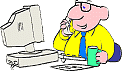1.Solve the system:(8,-2) (1,5) (4,2) (2,4) 2. Solve the system: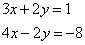(-1,2) (1,-1) (2,-1) (3,-5) 3. Solve the system: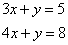(2,-1) (1,2) (3,-4) (1,4) 4. Solve the system: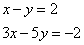(5/2,1/2) (6,4) (2, 0) (0,-2) 5. Solve the system: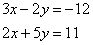(2,3) (3,1) (3,-2) (-2,3) 6. Solve the system:(-4,2) (-2,10) (-3,6) (2,4) 7. Solve the system: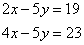(7,-1) (1,-1) (-3,2) (2,-3) 8. Solve the system: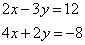(0,-4) (6,0) (3,-2) (1,-4) 9. Solve the system: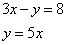(-2,-10) (1,5) (4,4) (-4,-20) 10. Solve the system:(-2,-5) (1,1) (-1,-3) (2,3) 11. Solve the system:(2,11) (7,31) (-2,5) (1,7) 12. Solve the system: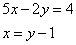(-4,-3) (2,3) (-1,0) (3,2) 13. Solve the system: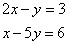(3, -3) (1,-1) (1,1) (-2,-7) 14. The sum of two numbers is 22. The larger is two less than twice the smaller. Find the larger. 10 17 14 9 15. One positive number is 7 more than 5 times another positive number.Their difference is 23. What is the smaller? 5 4 26 27 16. Three apples and two oranges cost \$3.80, while two apples and one orange costs \$2.30 Find the cost of an orange. \$.70 \$.80 \$1.50 \$.75 17. Trey wanted to cash a check for \$125 and asked the teller for only \$5 and \$10 bills. He got 16 bills. How many \$10 bills did he have? 7 10 9 6 18. 270 people paid a total of \$1065 to attend a recital. How many adults paid, if adult admission was \$5 and youth admission was \$2? 165 175 265 190